Q1.   Define the principal focus of a concave mirror.
Ans. It is a point on the principal axis where the rays of light parallel to principal axis meet.
Q2.   The radius of curvature of a spherical mirror is 20 cm. What is its fucul length?Q3.   Name a mirror that can give an erect and enlarged image of an object.
Ans. Concave mirror.
Q4.   Why do we prefer a convex mirror as a rear-view mirror in vehicles?
Ans. Convex mirror can cover a wider range and give erect and diminished image. Hence convex mirror is used as a rear-view mirror to get wider field of view.
Q5.   Find the focal length of a convex mirror whose radius of curvature is 32 cm.
Ans. Radius of curvature R = 32 cm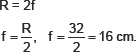Q6.   A concave mirror produces three times magnified (enlarged) real image of un object placed at 10cm in front of it. Where is the image located?
Ans. Object distance u = �10 cm (concave minor)Q7.   A ray of light travelling in cur enters obliquely into water. Does the light ray bend towards the normal or away form the normal? Why?
Ans. The light bends towards the normal on entry into water because water is optically denser than air.
Q8.   Light enters train air to glass having refractive index 1.50. What is the speed of light in the glass? The speed ref light in vacuum is 3 × 108 m/s.
Ans. The speed of light in vacuum is = 3 × 108 m/s
Refractive index of glass ng = 1.50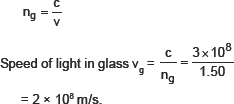Q9.   Find out from folllowing table the medium having highest optical derisity. Also find the medium with lowest optical density.Ans. Diamond has highest optical density i.e., 2.42 and air has the lowest optical density i.e., 1.0003.
Q10.   You are given kerosene, turpentine and water. In which of these does the light travel fastest? Use the information given in table above.
Ans. Refractive index of kerosene = 1.44
Refractive index of turpentine = 1.47
Refractive index of water = 1.33
Lower the refractive index faster is the speed of light in that medium. Hence light will travel fastest in water.
Q11.   The refractive index of diamond is 2.42. What is the meaning of this statement?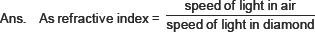This means the ratio of the speed of light in the air and the speed of light in diamond is equal to 2.42.
Q12.   Define I dioptre of newer of a lefts Ans. 1 dioptre is the power of a lens whose focal length is 1 metre.
1 D = 1 m�1
Q13.   A convex lens forms a real and inverted image of a needle at a distance of 50 cm from it. Where is the needle placed in front of the convex lens if the image is equal to the size of the object? Also find the power of the lens.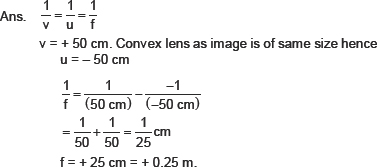Q14.   Find the power of a concave lens of focal length 2 m.
Ans. Focal length of concave lens f = �2 m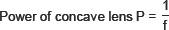QUESTIONS NCERT FROM TEXTBOOK
Q1.   Which one of the following rrratcr�ials cannot he used to make a lens?
(a) Water        (b) Glass
(c) Plastic        (d) Clay
Ans. (d) Clay
Q2.   The Image formed by a concave mirror is observed to be virtual, erect and larger than the object.
Where should he the positron of the object?
(a) Between the principal focus and the centre of carvature
(b) At the centre of curvature
(c) Beyond the centre of curvature
(d) Between the pole of the mirror and its principal focus
Ans. (d) Between the pole of the mirror and its principal focus.
Q3.   Where should an object be placed in front of a convex lens to get a real image of the size of the object?
(a) At the principal focus of the lens
(b) At twice the focal length
(c) At infinity
(d ) Between the optical centre of the lens and its principal focus
Ans. (b) At twice the focal length.
Q4.   A spherical mirror and a thin spherical lens have each a focal length of �15 cm. The mirror and the lens are likely to be
(a) both concave
(b) both convex
(c) the mirror is concave and the lens in convex.
(d) the mirror is convex but the lens is concave.
Ans. (a) Both concave.
Q5.   No matter how far stand from a mirror, your image appears erect. The mirror is likely to be
(a) plane.              (b) concave.
(c) convex           (d) either plane or convex.
Ans. (d) either plane or convex.
Q6.   Which of the following lenses would you prefer to use while reading small letters found in a dictionary?
(a) A convex lens of focal length 50 cm.
(b) A concave lens of focal length 50 cm.
(c) A convex- lens of focal length 5 cm.
(d) A concave lens of focal length 5 cm.
Ans. (c) A convex lens of focal length 5 cm.
Q7.   We wish to obtain an erect image of an object, using a concave minor of focal length 15 cm. What should he the range of distance of the object from the mirror? What is the nature of the image? Is the image larger or smaller than the object? Draw a ray diagram to show the image formation in this case.
Ans. Focal length of concave mirror = 15 cm.
Object should be place in front of given concave minor at a distance less than 15 cm.
Image formed is virtual and erect.
Image size is larger than object.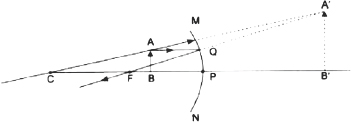Q8.   Name the type of mirror used in the following situations.
(a) Headlight of a car:           (b) Side/rear-view mirror of a vehicle
(c) Solar furnace.
Ans. (a) For headlight of a car- Concave mirror is used to get a powerful beam of light after reflection.(b) Convex minor is used for side/rear view mirror of a vehicle. Convex mirror forms an erect and diminished image of vehicles and gives wider view of rear.
(c) In solar furnace concave mirror is used as a reflector, it concentrates sun light at a point where the temperature increases sharply to 180°C � 200°C.
Q9.   One-half of a convex lens is covered with a black paper. Will this lens produce a complete image of the object? Verify your answer experimentally? Explain your observations.
Ans. Yes, one-half of a convex lens when covered with a black paper, the lens produces a complete or full image of an object.
To verify experimentally:
Take a convex lens, cover half part of it as shown in the figure, with a paper. Place it on a stand.
Focus a distant object on a screen, the image obtained on the screen is complete.
Observation and conclusion: Image formed on the screen does not depend on the size of the lens. The brightness of the image decreases as less number of rays pass through the lens.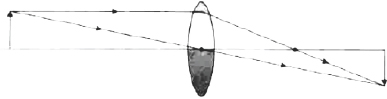Q10.   An object 5 cm in length is held 25 cm away from a converging lens of focal length 10 cm. Draw the ray diagram and find the position, size and the nature of the image formed.
Ans.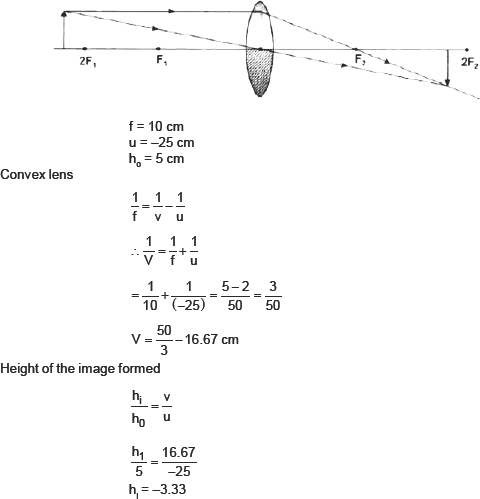Hence, the image formed at 16.67 cm from the lens on the other side. The size of the image is 3.3 cm, i.e., reduced and inverted.
Q11.   A concave lens of focal length 15 cm forms an image 10 cm from the lens object placed from the lens? Draw the ray diagram.
Ans. Concave lensQ12.   An object is placed at a distance of I0 cm from a convex mirror of focal length 15 cm. Find the position and nature of the image.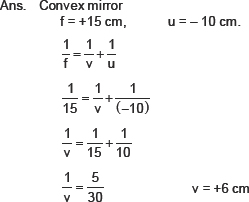The image is formed 6 cm behind the mirror, virtual image is formed.
Q13.   The magnification produced by a plane mirror is + 1. What does this mean?
Ans. Magnification,                      m = + 1
+ indicates virtual image.
1 indicates that the object size and image size is same.
Q14.   An object 5.0 cm in length is placed at a distance of 20 cm in front of a convex �error of radius of curvature 30 cm. Find the positron of the image, its nature and size.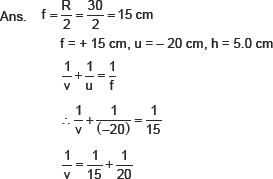Q15.   An object of size 7.0 cm is placed at 27 cm in front of a concave mirror of focal length 18 cm. At what distance from the mirror should a screen be placed, so that a sharp focussed image can he obtained? Find the size and the nature of the image.
Ans. Concave minor                  u = �27 cm, f = �18 cm, h = 7.0 cm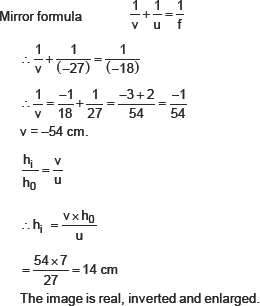Q16.   Find the focal length of a lens of power �2.0 D. What type of lens is this?
Ans. P = � 2.0 D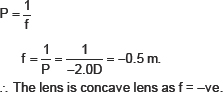Q17.   A doctor has prescribed a corrective lens of power +1.5 D. Find the focal length of the lens. Is the prescribed lens diverging or converging?Power of the lens is +ve, and it is converging lens i.e., convex lens.